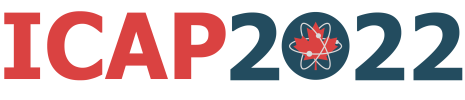#The 27th International Conference on Atomic Physics

Jul 17 – 22, 2022
Royal Conservatory of Music, Toronto
America/Toronto timezone

## Interferometry on the Clock Transition in Sr-87 with Entangled Atoms in Momentum State Superpositions

Jul 18, 2022, 5:00 PM
1h 30m
Hart House (Hart House)

### Hart House

#### Hart House

7 Hart House Cir, Toronto, ON M5S 3H3
Poster presentation Quantum optics and hybrid quantum systems

### Description

I will present our activity on the realisation of atom interferometry with an optical clock transition beyond the standard quantum limit (SQL) with strontium atoms. An interferometer can be injected with entangled atoms to improve its phase resolution $\Delta \phi$, where the entanglement is created between momentum state superpositions by performing cavity-enhanced quantum non-demolition (QND) measurements . Our method will improves the scaling in phase resolution $\Delta \phi$ with atom number $N$ surpassing the SQL of $\Delta \phi \sim N^{-1/2}$ for uncorrelated atoms. An interferometer using a similar method for entanglement creation has recently been demonstrated for a small atom number $N$ and a short interrogation time $T$ using rubidium atoms .

Atom interferometry can be used to study fundamental physics, like testing the equivalence principle or determining the gravitational constant $G$. It can furthermore be used in Earth observation sciences and in future space applications. To reach small phase resolutions $\Delta \phi$ interferometers need large-momentum transfer, a large atom number $N$ and a long interrogation time $T$. However, many applications are limited by the size, weight and power consumption of the instrument, this is especially true for space applications. An interferometer implementing QND measurements can therefore be reduced in size, weight and power consumption compared to interferometers using uncorrelated atoms. Furthermore, when using squeezing a given precision is reached after a smaller averaging time, which improves the sensor bandwidth.

We plan to create an atom interferometer on the clock transition ${^1S_0} \leftrightarrow {^3P_0}$ in $\text{strontium-87}$  and inject it with entangled atoms using QND measurements. We realised a narrow-linewidth clock laser system at 698 nm to drive the clock transition. We are working on a homodyne detection system to perform the QND measurements. A momentum state superposition will be created between ${\left\vert {^1S_0}, p = 0 \right\rangle}$ and ${\left\vert {^3P_0}, p = \hbar k \right\rangle}$ using single-photon transitions at 698 nm, with $\hbar k$ the momentum of a single photon. The QND measurements will be performed by probing the wave packets detuned from the ${^1S_0} \leftrightarrow {^3P_1}$ transition at 689 nm when they cross the focus of the high-finesse experimental cavity. Squeezing will be induced by measuring the populations in the prepared superposition. At the end of the interferometer sequence the population difference will be read out with increased sensitivity. This method can be used with other atomic species where a narrow transition is available or can be created.

 L. Salvi et al., Phys. Rev. Lett. $\textbf{120}$, 033601 (2018).
 G.P. Greve et al., arXiv $\textbf{2110}$, 14027v1 (2021).
 L. Hu et al., Class. Quantum Grav. $\textbf{37}$, 014001 (2020).

Presenter name Joep Assendelft

### Primary authors

Mr Joep Assendelft (European Laboratory for Non-linear Spectroscopy (LENS) and Dipartimento di Fisica e Astronomia) Dr Leonardo Salvi (European Laboratory for Non-linear Spectroscopy (LENS) and Dipartimento di Fisica e Astronomia) Dr Gabriele Rosi (European Laboratory for Non-linear Spectroscopy (LENS) and Dipartimento di Fisica e Astronomia) Dr Gunjan Verma (European Laboratory for Non-linear Spectroscopy (LENS) and Dipartimento di Fisica e Astronomia) Dr Enlong Wang (European Laboratory for Non-linear Spectroscopy (LENS) and Dipartimento di Fisica e Astronomia) Prof. Guglielmo Tino (European Laboratory for Non-linear Spectroscopy (LENS) and Dipartimento di Fisica e Astronomia)

### Presentation materials

There are no materials yet.